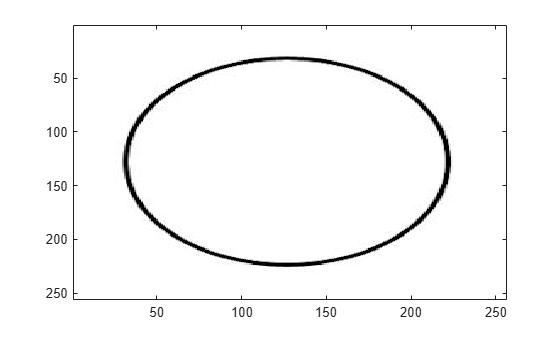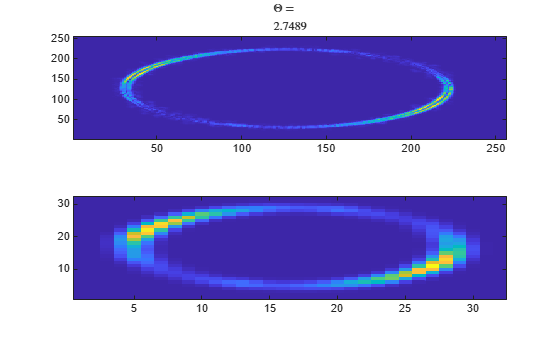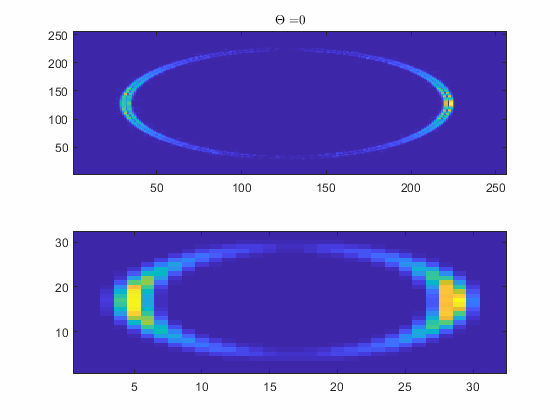Documentation

This is machine translation

Mouseover text to see original. Click the button below to return to the English version of the page.

scatteringTransform

Wavelet 2-D scattering transform

Syntax

``s = scatteringTransform(sf,im)``
``[s,u] = scatteringTransform(sf,im)``

Description

````s = scatteringTransform(sf,im)` returns the wavelet 2-D scattering transform of `im` for `sf`, the scattering decomposition framework. `im` is a real-valued 2-D matrix or 3-D matrix. If `im` is 3-D, the size of the third dimension must equal 3. The row and column sizes of `im` must match the `ImageSize` value of `sf`. The output `s` is a cell array with Nfb+1 elements, where Nfb is the number of filter banks in the scattering decomposition framework. Nfb is equal to the number of elements in the `QualityFactors` property of `sf`. Equivalently, the number of elements in `s` is equal to the number of orders in the scattering decomposition. Each element of `s` is a MATLAB® table.```

example

````[s,u] = scatteringTransform(sf,im)` also returns the wavelet scalogram coefficients for `im`. The output `u` is a cell array with Nfb+1 elements, where Nfb is the number of filter banks in the scattering decomposition framework. Nfb is equal to the number of elements in the `QualityFactors` property of `sf`. Equivalently, the number of elements in `u` is equal to the number of orders in the scattering decomposition. Each element of `u` is a MATLAB table.```

Examples

collapse all

This example shows that scattering coefficients are lowpassed versions of scalogram coefficients.

Load an RGB image. Display the red channel.

```im = imread('circle.jpg'); size(im)```
```ans = 1×3 256 256 3 ```
```figure imagesc(im(:,:,1)) colormap gray;```For RGB images, the size of the third dimension must be 3. You only have to specify the row and column sizes of the image when you create the scattering framework. Create a scattering framework to apply to the image and take the scattering transform.

```sf = waveletScattering2('ImageSize',[256 256],'InvarianceScale',32,... 'NumRotations',[8 8]); [S,U] = scatteringTransform(sf,im);```

The image and coefficient fields in `S` and `U` are M-by-N-by-3. The M-by-N dimensions are constant only in the scattering images because the scaling function has fixed bandwidth, while the wavelets have different bandwidths.

Use a for-loop and plot the red channel for the scalogram and scattering coefficients for the 8 rotation angles in the scattering transform. Note how the scattering coefficients are lowpass versions of the scalogram coefficients.

```[~,~,~,filterparams] = sf.filterbank(); theta = filterparams{1}.rotations; figure for k = 1:numel(theta) subplot(2,1,1) imagesc(U{2}.coefficients{k}(:,:,1)); axis xy title(['\$\$\Theta = \$\$' num2str(theta(k))],'Interpreter','Latex'); subplot(2,1,2) imagesc(S{2}.images{k}(:,:,1)); axis xy pause(1) end```The above for-loop results in an animation identical to the one below.Input Arguments

collapse all

Scattering decomposition framework, specified as a `waveletScattering2` object.

Input image, specified as a real-valued 2-D matrix or 3-D matrix. If `im` is 3-D, `im` is assumed to be a color image in the RGB color space, and the size of the third dimension must equal 3. The row and column sizes of `im` must match the `ImageSize` property of `sf`.

Output Arguments

collapse all

Scattering coefficients, returned as a cell array. `s` is a cell array with Nfb+1 elements where Nfb is the number of filter banks in the scattering decomposition framework. Nfb is equal to the number of elements in the `QualityFactors` property of `sf`. Equivalently, the number of elements in `s` is equal to the number of orders in the scattering decomposition. Each element of `s` is a MATLAB table with these variables:

Scattering coefficients, returned as a cell array. Each element of `images` is an M-by-N or M-by-N-by-3 matrix.

Scattering path used to obtain the scattering coefficients, returned as a row vector. Each column of `path` corresponds to one element of the path. The scalar 0 denotes the original image. Positive integers in the Lth column denote the corresponding wavelet filter in the (L−1)th filter bank. Wavelet bandpass filters are ordered by decreasing center frequency.

There are `NumRotations` wavelets per center frequency pair.

Bandwidth of scattering coefficients, returned as a scalar. The bandwidth is symmetric in the x and y directions.

Base-2 log resolution of the scattering coefficients, returned as a scalar.

Scalogram coefficients, returned as a cell array. `u` is a cell array with Nfb+1 elements, where Nfb is the number of filter banks in the scattering decomposition framework. Nfb is equal to the number of elements in the `QualityFactors` property of `sf`. Equivalently, the number of elements in `u` is equal to the number of orders in the scattering decomposition. Each element of `u` is a MATLAB table with these variables:

Scalogram coefficients, returned as a cell array. Each element of `coefficients` is an M-by-N or M-by-N-by-3 matrix.

Scattering path used to obtain the scalogram coefficients, returned as a row vector. Each column of `path` corresponds to one element of the path. The scalar 0 denotes the original image. Positive integers in the Lth column denote the corresponding wavelet filter in the (L−1)th filter bank. Wavelet bandpass filters are ordered by decreasing center frequency.

There are `NumRotations` wavelets per center frequency pair.

Bandwidth of scalogram coefficients, returned as a scalar.

Base-2 log resolution of the scattering coefficients, returned as a scalar.

Introduced in R2019a

Wavelet Toolbox Documentation